Start typing, then use the up and down arrows to select an option from the list.# Molar Solubility of Ions

Jules Bruno
175views
2
so an example to they tell us that calcium phosphate has a K S p up 5.8 times 10 to the negative 32. Or ask what is the Moller Saudi ability of calcium ions in a saturated solution off calcium phosphate? Since we're dealing with K SP, we're talking about the ionic solid form of this and how it breaks up into its ions. So breaks up into calcium ions and there are three calcium ions. Remember, Cal seems a group two ways, so that's why it's plus two. Then it breaks up into two phosphate ions, which is a poly atomic ion. Since we're dealing with K SP, we're dealing with a nice chart. Okay? And remember, in a nice chart, we ignore solids and liquids. Here. This is breaking up in pure water. So these two are initially zero Here there are three calcium. So this is three X. There are two phosphates. So this is two X So this is plus three x plus two x Remember, k SP will equal products here. So it's calcium ion. Remember, the coefficients become powers phosphate ion here. So now we plug in what we know. So K s P here is 58 times 10 to the negative 32. So this is three X cubed. This is two X squared. So three cubed is three times three times three, which is 27 and two squared is four. So now we have 27 times four, which is 108 and then X to the third times X to the second you add the exponents. So that's X to the fifth and this equals my K sp, which is still 5.8 times 10 to the negative. 32. We need toe isolate X. So we're gonna divide both sides here by So X to the fifth equals 5. times 10 to the negative 34. Now you may see this bun on your calculator. So you do five. You hit this button, then put 537 times 10 to the negative, 34 in parentheses. If not, you might see this button or this fun instead. So you would do this number in parentheses and then it also in parentheses. After you hit this number, this butter this button you put in parentheses one to the fifth to help you take the fifth root. So when we do that, that would give me my ex. Which comes out to 222 times 10 to the negative seven Moeller. But this X represents the Saudi ability of the entire Ionic compound, but we don't want the Saudi ability, the entire Ionic compound. We want the Mueller Saudi ability of calcium ions. So we look on the equilibrium line for calcium and we see that of equals three x. So we take this X and plug it in here so you multiply it by three. So that comes out to 6.7 times 10 to the negative seven Mueller. So this will be the concentration or Moeller Salyer bility of calcium ions. Remember, if we want the Mueller saw ability of the entire compound, it's just X. But if we want the Mueller Saudi ability of a particular ion, we look at the equilibrium line and we plug in the answer for X that we got in for that ion. If we wanted phosphate, then we plug that X into here to find the Mueller saw the ability of phosphate ions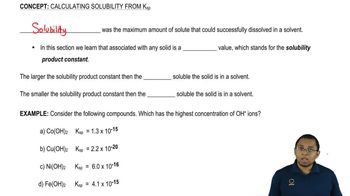01:30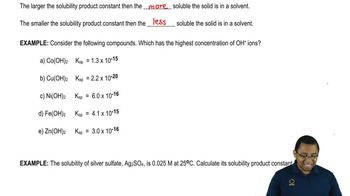01:5905:51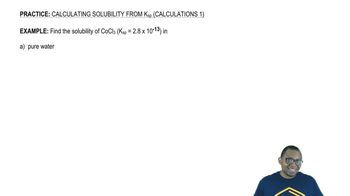05:2104:1104:5005:15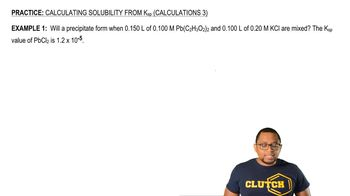07:1606:0503:47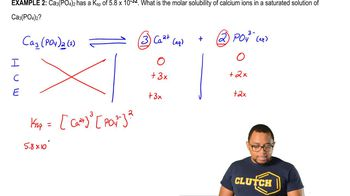04:1003:44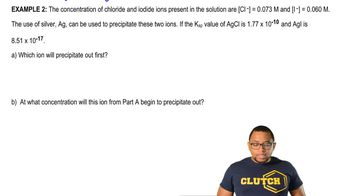03:36